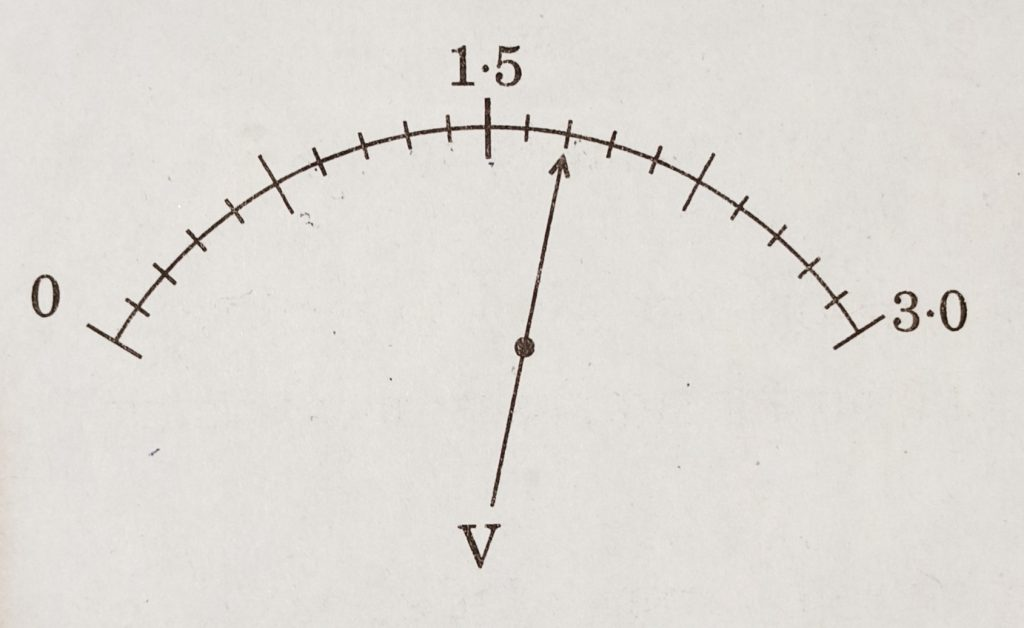# Class 10 Electricity Previous Years Questions

Electricity Previous Years Questions

1. Define 1 kilowatt hour. [1 mark] [CBSE 2019]
2. The values of mA and µA are: [1 mark] [CBSE 2020]
1. 10-6 A and 10-9 A respectively
2. 10-3 A and 10-6 A respectively
3. 10-3 A and 10-9 A respectively
4. 10-6 A and 10-3 A respectively
3. A cylindrical conductor of length ‘l’ and uniform area of cross-section ‘A’ has resistance ‘R’. Another conductor of length 2.5 l and resistance 0.5 R of the same material has area of cross-section: [1 mark] [CBSE 2020]
1. 5 A
2. 2.5 A
3. 0.5 A
4. 1/5 A
4. Define the term electrical resistivity of a material. [1 mark] [CBSE 2019]
5. Consider the scale of a voltmeter shown in the diagram and answer the following questions:1. What is the least count of the voltmeter?
2. What is the reading shown by the voltmeter?
3. If this voltmeter is connected across a resistor of 20 Ω, how much current is flowing through the resistor. [2 marks] [CBSE 2019]
6. The values of current (I) flowing through a given resistor (R), for the corresponding values of potential difference (V) across the resistor are as given below :

Plot a graph between current (I) and potential difference (V) and determine the resistance (R) of the resistor. [2 marks] [CBSE 2018]

7. Show how would you join three resistors each of resistance 9 Ω so that the equivalent resistance of the combination is (i) 13.5 Ω (ii) 6 Ω? [3 marks] [CBSE 2018]

8. a. Write Joule’s law of heating.
b. Two lamps, one rated 100 W; 220 V, and the other 60 W; 220 V, are connected in parallel to electric mains supply. Find the current drawn by two bulbs from the line, if the supply voltage is 220 V. [3 marks] [CBSE 2018]

9. a. List the factors on which the resistance of a conductor in the shape of a wire depends.
b. Why are metals good conductor of electricity whereas glass is a bad conductor of electricity ?Give reason.
c. Why are alloys commonly used in electrical heating devices? Give reason. [CBSE 2018] [3 Marks]

10. a. Three resistors of resistances R1, R2 & R3 are connected (i) in series , & (ii) in parallel. Write expressions for the equivalent resistance of the combination in each case.
b. Two identical resistors of 12 Ω each are connected to a battery of 3 V. Calculate the ratio of the power consumed by the resulting combinations with minimum resistance & maximum resistance. [CBSE 2019] [5 Marks]

11. a. Write the relation between resistance & electrical resistivity of the material of a conductor in the shape of a cylinder of length ‘l’ & area of cross section ‘A’ . Hence derive the S.I. unit of electrical resistivity.
b. Resistance of a metal wire of length 5 m is 100 Ω. If the area of cross section of the wire is 3 x 10-7 m2, calculate the resistivity of the metal. [CBSE 2019] [5 Marks]

12. a. Two lamps rated 100 W, 220 V& 10 W , 220 V are connected in parallel to 220 V supply , calculate the total current through the circuit.
b. Two resistors x & y of resistances 2 Ω & 3 Ω respectively are first joined in parallel & then in series . In each case the voltage supplied is 5 V.
(i) Draw circuit diagrams to show the combination of resistors in each case.
(ii) Calculate the voltage across the 3 Ω resistor in the series combination of resistors. [CBSE 2020] [5 Marks]

13. a. State ohm’s law.
b. How is an ammeter connected in an electric circuit?
c. The power of a lamp is 100 W. Find the energy consumed by it in 1 minute.
d. A wire of resistance 5 Ω is bent in the form of a closed circle. Find the resistance between two points at the ends of any diameter of the circle. [5 marks] [CBSE 2020]